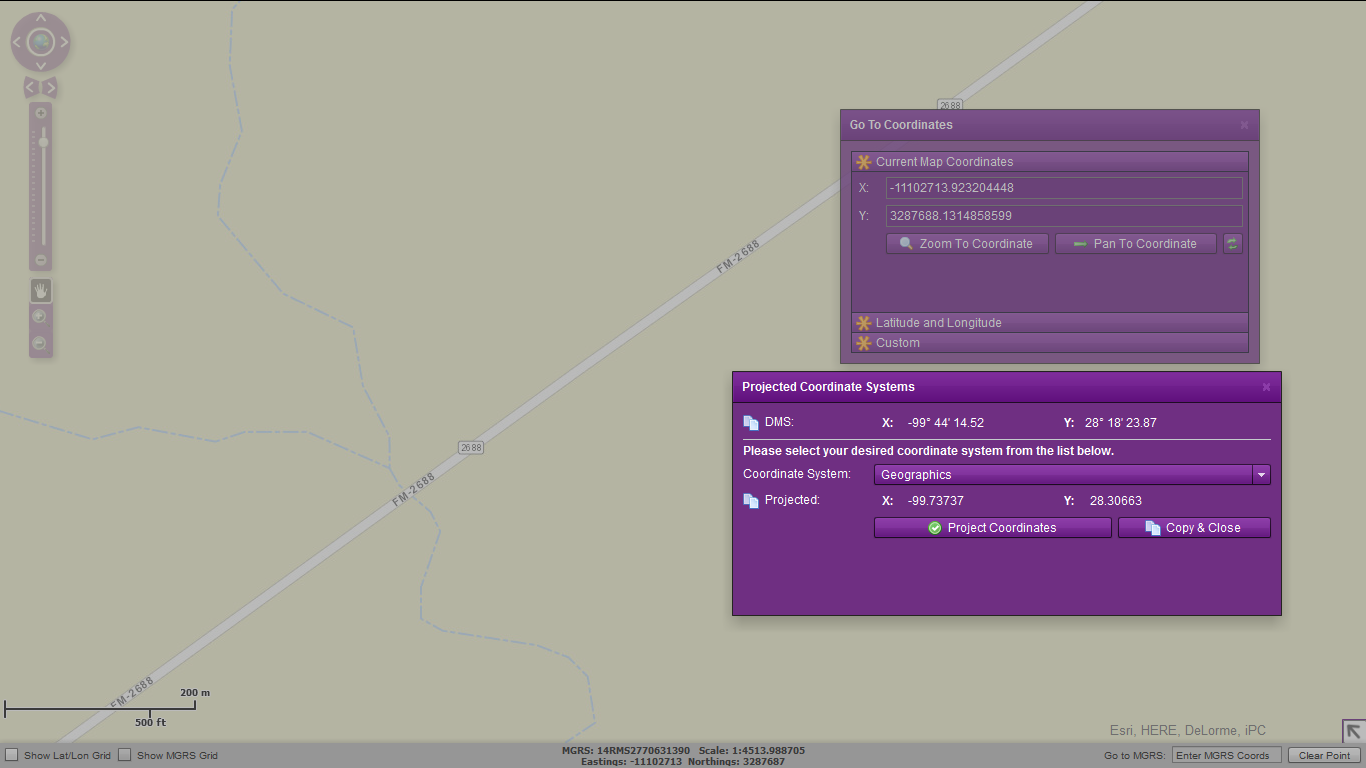# What coordinate is this and how can I convert it?

1744
7
07-23-2014 01:27 PM
Highlighted
New Contributor

I have a coordinate database using coordinates like this: 3288396.9786247308, -11102996.686625781

I used a random website (Coordinate Menu Widget) to look it up, and it went right where it was supposed to, so it must be fairly universal.

My first question is, what is the name of this system so I can learn how to convert it? I thought it was UTM but I haven't been able to convert it with any online tools.

Second, do you know of any programming libraries that convert this (PHP, JS, Java, C#, etc.)? Preferably PHP.

1 Solution

Accepted Solutions
Highlighted
Frequent Contributor II

The chances are that it is in web mercator auxiliary sphere then as that is the coordinate system esri use for its basemaps. The maths for the conversion can be found on this blog:

7 Replies
Highlighted
Frequent Contributor

sounds like a good one for Melita Kennedy

Highlighted
Frequent Contributor II

It could be web Mercator, what was the source of the database?

Highlighted
New Contributor

It is from an ESRI viewer. The JSON says "`esriGeometryPoint`".

I meant to say earlier that the point given goes to a spot in Texas. The lat/lng should be around 28,-99.

Highlighted
Frequent Contributor II

The chances are that it is in web mercator auxiliary sphere then as that is the coordinate system esri use for its basemaps. The maths for the conversion can be found on this blog:

Highlighted
New Contributor

I actually just stumbled upon that method and converted it to PHP, but it's wrong. Anyone able to see why it didn't work? Maybe it's not web mercator? Or I failed at converting it?

function convert(\$mercatorX_lon,\$mercatorY_lat) {

\$x = \$mercatorX_lon;

\$y = \$mercatorY_lat;

\$num3 = \$x / 6378137.0;

\$num4 = \$num3 * 57.295779513082323;

\$num5 = floor((\$num4 + 180.0) / 360.0);

\$num6 = \$num4 - (\$num5 * 360.0);

\$num7 = 1.5707963267948966 - (2.0 * atan(log ((-1.0 * \$y) / 6378137.0)));

\$mercatorX_lon = \$num6;

\$mercatorY_lat = \$num7 * 57.295779513082323;

return \$mercatorX_lon.','.\$mercatorY_lat;

}

echo convert(3287688.1314858599,-11102713.923204448);

// returns: 29.5338049793,31.9996076332

Actual coordinates: 28.3063916, -99.7370334

Highlighted
Frequent Contributor II

I think it is definitely web mercator as my flex application is converting correctly, the only thing i can see which may be causing an issue is num 5, the code specifically floors to a double:

double num5 = Math.Floor((double)((num4 + 180.0) / 360.0));

where yours is not:

\$num5 = floor((\$num4 + 180.0) / 360.0);

This maybe causing some rounding somewhere?Highlighted
New Contributor

Anthony, thanks for the help! I found my mistake. I grabbed the wrong PHP function for exp. It was just the same as java (exp). I also put it in backwards, ouch... long day!

For those who need it, the PHP function for converting web mercator to latitude longitude is:

function convert(\$mercatorX_lon,\$mercatorY_lat) {

\$x = \$mercatorX_lon;

\$y = \$mercatorY_lat;

\$num3 = \$x / 6378137.0;

\$num4 = \$num3 * 57.295779513082323;

\$ex = (\$num4 + 180.0) / 360.0;

\$num5 = floor(\$ex); // Math.Floor((num4 + 180.0) / 360.0);

//echo \$num5 .'and'.\$ex; die;

\$num6 = \$num4 - (\$num5 * 360.0);

\$num7 = 1.5707963267948966 - (2.0 * atan(exp ((-1.0 * \$y) / 6378137.0)));

//echo \$num6 . ' and ' .\$num7; die;

\$mercatorX_lon = \$num6;

\$mercatorY_lat = \$num7 * 57.295779513082323;

return \$mercatorX_lon.','.\$mercatorY_lat;

}